Checkout JEE MAINS 2022 Question Paper Analysis : Checkout JEE MAINS 2022 Question Paper Analysis :

# I.E. Irodov Solutions on Relativistic Mechanics

The Solutions of the Problems In General Physics I.E. Irodov - Relativistic Mechanics are given on this page. Relativistic mechanics is an important topic in the physical fundamentals of mechanics. The main concepts in this topic are Lorentz contraction of length and slowing of a moving clock, Lorentz transformation, Transformation of velocity, Relativistic mass, and relativistic momentum, etc. It also includes relativistic equation of dynamics for a particle and total and kinetic energies of a relativistic particle. Students can expect one question from this topic.

Students are advised to learn these solutions so that they can easily score higher marks in the JEE Main and other entrance exams.

### I.E. Irodov Solutions on Relativistic Mechanics

1. A rod moves lengthwise with a constant velocity v relative to the inertial reference frame K. At what value of v will the length of the rod in this frame be η = 0.5% less than its proper length?

Solution:

1. From the formula for length contraction

(l0 - l0√(1 - v2/c2)) = ηl0

So 1 - v2/c2 = (1 - η)2

Or v = c√(η (2 - η))

2. In a triangle the proper length of each side equals a. Find the perimeter of this triangle in the reference frame moving relative to it with a constant velocity V along one of its

1. (a) bisectors;

2. (b) sides.

Solution:

1. (a) In the frame in which the triangle is at rest the space coordinates of the vertices are (0, 0 , 0), (a√3/2, a/2, 0), (a√3/2, -a/2, 0), all measured at the same time t. In the moving frame the corresponding coordinates at time t’ are

A: (vt’, 0, 0), B: ((a√3/2)√(1 - β2) + vt’, a/2, 0) and C: ((a/2)√3√(1 - β2) + vt’, -a/2, 0)

The perimeter P is then

P = a + 2a(¾ (1 - β2) + ¼)1/2

= a(1 + √(4 - 3β2)

(b) The coordinates in the first frame are shown at time t. The coordinates in the moving frame are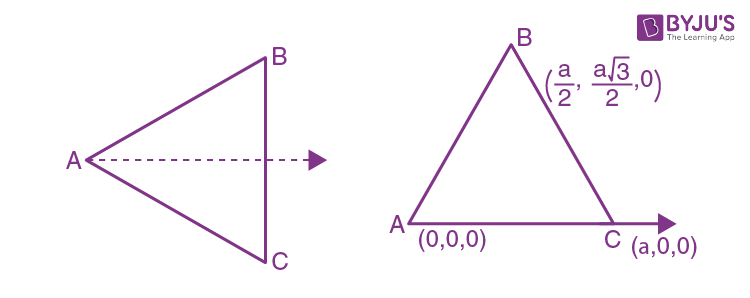A: (vt’, 0, 0), B: ((a/2)√(1 - β2) + vt’, a√3/2, 0), C: (a√(1 - β2) + vt’, 0, 0)

The perimeter P is then

P = a√(1 - β2) + (a/2)(1 - β2 + 3)1/2 × 2 = a(√(1 - β2) + √(4 - β2)) here β = V/c

3. Find the proper length of a rod if in the laboratory frame of reference its velocity is v = c/2, the length l = 1.00 m, and the angle between the rod and its direction of motion is θ = 450.

Solution:

1. In the rest frame, the coordinates of the ends of the rod in terms of proper length l0

A: (0, 0, 0) B: (l0 cos θ0, l0 sin θ0, 0) at time t. In the laboratory frame the coordinates at time t’ are A: (vt’, 0, 0), B: (l0 cos θ0√(1 - β2) + vt’, l0 sin θ0, 0)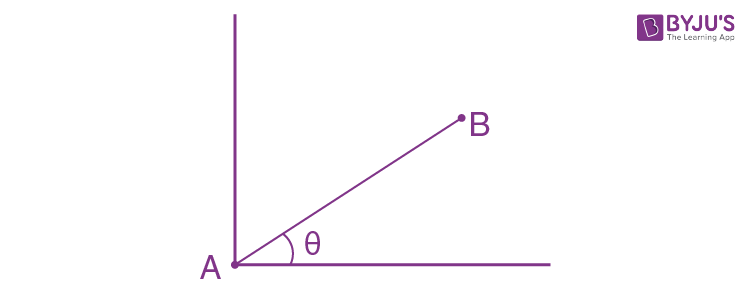Therefore, we can write

l cos θ0 = l0 cos θ0√(1 - β2) and l sin θ = l0 sin θ0

Hence l02 = l2 (cos2θ + (1 - β2) sin2θ)/(1 - β2)

Or = √[(1 - β2 sin2θ)/(1 - β2)]

4. A stationary upright cone has a taper angle 0 =. 45°, and the area of the lateral surface So = 4.0 m2. Find: (a) its taper angle; (b) its lateral surface area, in the reference frame moving with a velocity v = (4/5)c along the axis of the cone.

Solution:

1. In the frame K in which the cone is at rest the coordinates of A are (0, 0, 0) and of B are (h, h tan θ, 0). In the frame K’, which is moving with velocity v along the axis of the cone, the coordinates of A and B at time t’ are

A : (-vt’, 0, 0), B(h√(1 - β2) - vt’, h tan θ, 0)

Thus the taper angle in the frame K’ is tan θ’ = tan θ/√(1 - β2)

= (y’B - y’A)/(x’B - x’A)

And the lateral surface area is,

S = πh’2sec θ’ tan θ’

= πh2(1 - β2) (tan θ/√(1 - β2))(√(1 + tan2 θ/(1 - β2)

= S0√(1 - β2 cos2θ)

Here S0 = πh2 sec θ tan θ is the lateral surface area in the rest frame and

h’ = h√(1 - β2), β = v/c

5. With what velocity (relative to the reference frame K) did the clock move, if during the time interval t = 5.0 s, measured by the clock of the frame K, it became slow by ∆t = 0.10 s?

Solution:

1. Because of time dilation, a moving clock reads less time.

We write, t - ∆t = t√(1 - β2), β = v/c

Thus 1 - 2∆t/t + (∆t/t)2 = 1 - β2

Or v = c√(∆t/t)(2 - ∆t/t)

6. A rod flies with constant velocity past a mark which is stationary in the reference frame K. In the frame K it takes ∆t = 20 ns for the rod to fly past the mark. In the reference frame fixed to the rod, the mark moves past the rod for ∆t’ = 25 ns. Find the proper length of the rod.

Solution:

1. In the frame K the length l of the rod is related to the time of flight ∆t by

l = v∆t

In the reference frame fixed to the rod (frame K') the proper length l0 of the rod is given by

l0 = v∆t’

But l0 = l/√(1 - β2)

= v∆t/√(1 - β2)

β = v/c

Thus, v∆t’ = v∆t/√(1 - β2)

So (1 - β2) = (∆t/∆t’)2

Or v = c√(1 -(∆t/∆t’)2)

And l0 = c√(∆t’)2 - (∆t)2

= c∆t’/√(1 - (∆t/∆t’)2)

7. The proper lifetime of an unstable particle is equal to ∆t0= 10 ns. Find the distance this particle will traverse till its decay in the laboratory frame of reference, where its lifetime is equal to ∆t = 20 ns.

Solution:

1. The distance traveled in the laboratory frame of reference is v∆t where v is the velocity of the particle. But by time dilation

∆t = ∆t0/√(1 - v2/c2)

So v = c√(1 - (∆t0/∆t)2

Thus the distance traversed is c∆t√(1 - (∆t0/∆t)2

8. In the reference frame K a muon moving with a velocity v = 0.990c travelled a distance l = 3.0 km from its birthplace to the point where it decayed. Find:

1. (a) the proper lifetime of this muon;

2. (b) the distance travelled by the muon in the frame K “from the muon's standpoint”.

Solution:

1. If T0 is the proper life time of the muon the lifetime in the moving frame is

T0/√(1 - v2/c2) and hence l = vT0/√(1 - v2/c2)

Thus T0 = (l/v)√(1 - v2/c2)

(The words "from the muon’s stand point" are not part of any standard terminology)

9. Two particles moving in a laboratory frame of reference along the same straight line with the same velocity v = (3/4)c strike against a stationary target with the time interval ∆t = 50 ns. Find the proper distance between the particles prior to their hitting the target.

Solution:

1. In the frame K in which the particles are at rest, their positions are A and B whose coordinates may be taken as,

A: (0, 0, 0) B = (l0, 0, 0)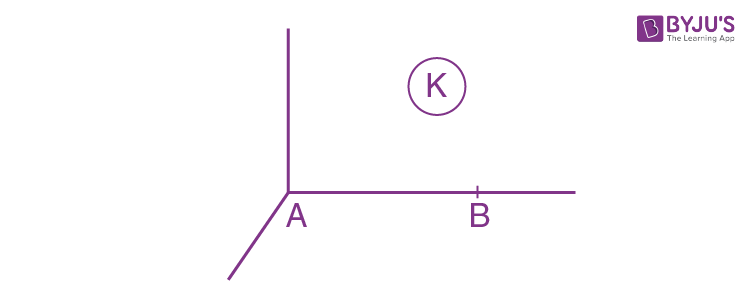In the frame K’ with respect to which K is moving with a velocity v the coordinates of A and B at time t' in the moving frame are

A = (vt’, 0, 0)

B = (l0√(1 - β2) + vt’, 0, 0), β = v/c

Suppose B hits a stationary target in K’ after time t’B while A hits it after time tB + ∆t. Then

l0√(1 - β2) + vt’B = v(t’B + ∆t)

So l0 = v∆t/√(1 - v2/c2)

10. A rod moves along a ruler with a constant velocity. When the positions of both ends of the rod are marked simultaneously in the reference frame fixed to the ruler, the difference of readings on the ruler is equal to ∆x1= 4.0 m. But when the positions of the rod's ends are marked simultaneously in the reference frame fixed to the rod, the difference of readings on the same ruler is equal to ∆x2 = 9.0 m. Find the proper length of the rod and its velocity relative to the ruler.

Solution:

1. In the reference frame fixed to the ruler, the rod is moving with a velocity v and suffers Lorentz contraction. If l0 is the proper length of the rod, its measured length will be

∆x1 = l0√(1 - β2), β = v/c

In the reference frame fixed to the rod the ruler suffers Lorentz contraction and we must have

∆x2 √(1 - β2) = l0

Thus l0 = √(∆x1∆x2)

And 1 - β2 = ∆x1/∆x2

Or v = c√(1 - ∆x1/∆x2)

11. Two rods of the same proper length l0 move toward each other parallel to a common horizontal axis. In the reference frame fixed to one of the rods the time interval between the moments, when the right and left ends of the rods coincide, is equal to Δt. What is the velocity of one rod relative to the other?

Solution:

1. The coordinates of the ends of the rods in the frame fixed to the left rod are shown. The points B and D coincides when

l0 = c1 - vt0 or t0 = (c1 - l0)/v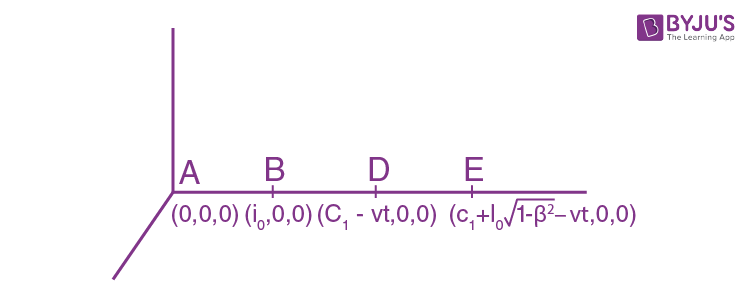The points A and E coincide when

0 = c1 = l0√(1 - β2) - vt1, t1 = (c1 + l0√(1 - β2)/v)

Thus ∆t = t1 - t0

= (l0/v)(1 + √(1 - β2))

Or (v∆t/l0 - 1)2 = (1 - β2)

= 1 - v2/c2

From this v = 2c2∆t/l0/(1 + c2∆t2/l02)

= 2l0/∆t/(1 + (l0/c∆t)2)

12. Two unstable particles move in the reference frame K along a straight line in the same direction with a velocity v = 0.990c. The distance between them in this reference frame is equal to l = 120 m. At a certain moment, both particles decay simultaneously in the reference frame fixed to them. What time interval between the moments of decay of the two particles will be observed in the frame K? Which particle decays later in the frame K?

Solution:

1. In K0 , the rest frame of the particles, the events corresponding to the decay of the particles are,

A:(0, 0, 0) and (0, l0, 0, 0) = B

In the reference frame K, the corresponding coordinates are by Lorentz transformation

A:(0,0, 0), B: (vl0/c2√(1 - β2), l0/√(1 - β2), 0, 0)

Now l0√(1 - β2) = l

By Lorentz Fitzgerald contraction formula. Thus the time lag of the decay time of B is

∆t = vl0/c2√(1 - β2)

= vl/c2(1 - β2)

= vl/(c2 - v2)

B decays later (B is the forward particle in the direction of motion)

13. A rod AB oriented along the x-axis of the reference frame K moves in the positive direction of the x-axis with a constant velocity v. The point A is the forward end of the rod, and the point B its rear end. Find:

1. (a) the proper length of the rod, if at the moment tA the coordinate of the point A is equal to xA, and at the moment tB the coordinate of the point B is equal to xB

2. (b) what time interval should separate the markings of coordinates of the rod’s ends in the frame K for the difference of coordinates to become equal to the proper length of the rod.

Solution:

1. (a) In the reference frame K with respect to which the rod is moving with velocity v, the coordinates of A and B are

A: t, xA + v(t - tA), 0, 0

B: t, xB + v(t - tB), 0, 0

Thus l = xA - xB - v(tA - tB)

= l0√(1 - β2)

So l0 = (xA - xB - v(tA - tB)/√(1 - v2/c2)

(b) ±l0 - v(tA- tB)= l = l0√(1 - v2/c2)

(Since xA - xB can be either + l0 or - l0)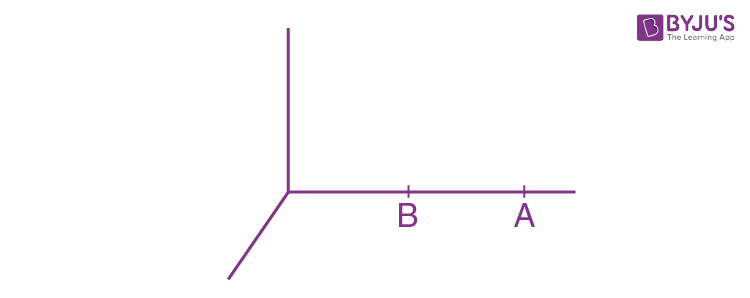Thus v(tA - tB) = ±1 - √(1 - v2/c2)l0

I.e, tA - tB = (l0/v)(1 - √(1 - v2/c2)

Or tB - tA = (l0/v)(1 + √(1 - v2/c2)

14. The rod A'B' moves with a constant velocity v relative to the rod AB (Fig. 1.91). Both rods have the same proper length l0 and at the ends of each of them clocks are mounted, which are synchronized pairwise: A with B and A' with B'. Suppose the moment when the clock B' gets opposite the clock A is taken for the beginning of the time count in the reference frames fixed to each of the rods. Determine:

1. (a) the readings of the clocks B and B' at the moment when they are opposite each other;

2. (b) the same for the clocks A and A'.

Solution:

1. At the instant the picture is taken the coordinates of A, B, A’, B’ in the rest frame of AB are

A: (0, 0, 0, 0)

B: (0, l0, 0, 0)

B’: (0, 0, 0, 0)

A’ : (0 - l0√(1 - v2/c2), 0, 0)

In this frame the coordinates of B’ at other times are B': (t, vt, 0, 0). So B' is opposite to B at time t(B) = l0/v. In the frame in which B', A' is at rest the time corresponding this is by Lorentz transformation

t0(B’) = (1/√(1 - v2/c2))(l0/v - vl0/c2)

= (l0/v)√(1 - v2/c2)

Similarly in the rest frame of A, B, the coordinates of A at other times are

A’ : (t, -l0√(1 - v2/c2) + vt, 0, 0)

A’ is opposite to A at time t(A) = (l0/v)√(1 - v2/c2)

The corresponding time in the frame in which A’, B’ are at rest is

t(A’) = γt(A)

= l0/v

15. There are two groups of mutually synchronized clocks K and K' moving relative to each other with a velocity v as shown in Fig. 1.92. The moment when the clock A' gets opposite the clock A is taken for the beginning of the time count. Draw the approximate position of hands of all the clocks at this moment "in terms of the K clocks"; "in terms of the K' clocks".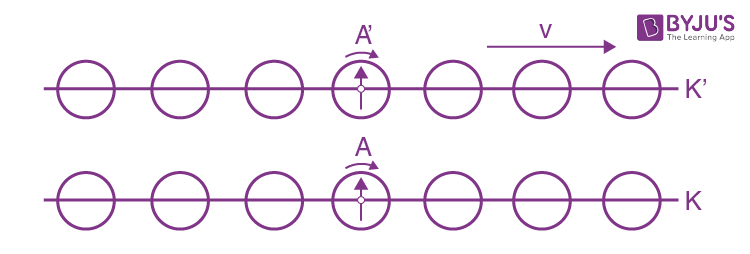Solution:

1. By Lorentz transformation t’= (1/√(1 - v2/c2))(t - vx/c2)

So at time t = 0, t’ = (vx/c2)1/√(1 - v2/c2)

If x>0, t’<0, if x<0, t’>0 and we get the diagram given below in terms of the K - clock.The situation in terms of the K’ clock is reversed.

16. The reference frame K' moves in the positive direction of the x-axis of the frame K with a relative velocity V. Suppose that at the moment when the origins of coordinates O and O' coincide, the clock readings at these points are equal to zero in both frames. Find the displacement velocity x of the point (in the frame K) at which the readings of the clocks of both reference frames will be permanently identical. Demonstrate that x < V.

Solution:

1. Suppose x(t) is the locus of points in the frame K at which the readings of the clocks of both reference system are permanently identical, then by Lorentz transformation

t’ = 1/√(1 - V2/c2)

= (c/β)(1 - √(1 - β2)

β = V/c

Let β = tan hθ, 0≤ θ< ∞, then

x(t) = (c/tan hθ)(1 - √(1 - tan h2θ)

= c (cos hθ/sin hθ)(1 - 1/cos hθ)

= c√(cos hθ - 1)/(cos hθ + 1)

= c tan h θ/2 ≤ v

(tan h θ is a monotonically increasing function of θ)

17. At two points of the reference frame K two events occurred separated by a time interval At. Demonstrate that if these events obey the cause-and-effect relationship in the frame K (e.g. a shot fired and a bullet hitting a target), they obey that relationship in any other inertial reference frame K’.

Solution:

1. We can take the coordinates of the two events to be

A: (0, 0, 0, 0) B: (∆t, a, 0, 0)

For B to be the effect and A to because we must have ∆t > |a|/c.

In the moving frame the coordinates of A and B become

A: (0, 0, 0, 0), B: (γ(∆t = aV/c2), γ(a - V∆t), 0, 0) where γ = 1/√(1 - V2/c2)

Since

(∆t’)2 - a’2/c2 = γ2(∆t - aV/c2) - 1/c2)(a - V∆t)2

= (∆t)2 - a2/c2 > 0

We must have ∆t’ > |a’|/c

18. The space-time diagram of Fig. 1.93 shows three events A, B, and C which occurred on the x axis of some inertial reference frame. Find:

1. (a) the time interval between the events A and B in the reference frame where the two events occurred at the same point;

2. (b) the distance between the points at which the events A and C occurred in the reference frame where these two events are simultaneous.

Solution:

1. The four dimensional interval between A and B (assuming Δy = Δz = 0) is

52 - 32 = 16 units

Therefore the time interval between these two events in the reference frame in which the events occurred at the same place is

c(t’B - t’A) = √16 = 4 m

(t’B - t’A) = 4/c = (4/3)×10-8 s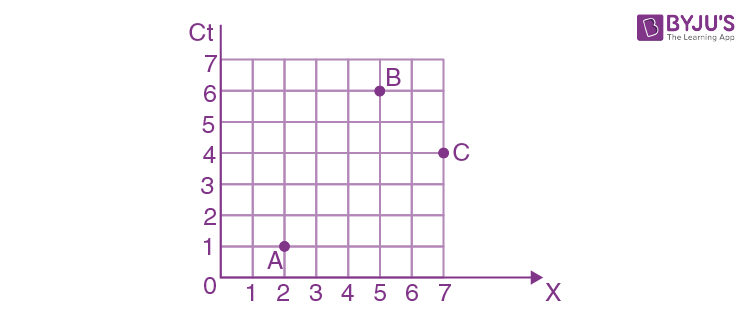(b) The four dimensional interval between A and C is (assuming Δy = Δz = 0)

32 - 52 = -16

So the distance between the two events in the frame in which they are simultaneous is 4 units = 4m.

19. The velocity components of a particle moving in the xy plane of the reference frame K are equal to vx and v y . Find the velocity v’ of this particle in the frame K' which moves with the velocity V relative to the frame K in the positive direction of its x axis.

Solution:

1. By the velocity addition formula

vx= (vx - V)/(1 - Vvx/c2)

v’y = vy √(1 - V2/c2)/(1 - vxV/c2)

And v’ = √(v’x2 + v’y2)

= √[(vx - V)2 + vy2(1 - V2/c2)]/1 - vxV/c2

20. Two particles move toward each other with velocities v1 = 0.50c and v2 = 0.75c relative to a laboratory frame of reference.

Find:

1. (a) the approach velocity of the particles in the laboratory frame of reference;

2. (b) their relative velocity.

Solution:

1. (a) By definition the velocity of approach is

vapproach = dx1/dt - dx2/dt

= v1 - (-v2)

= v1 + v2

in the reference frame K.

(b) The relative velocity is obtained by the transformation law

vr = (v1 -(-v2)/(1 - v1(-v2)/c2

= (v1 + v2)/(1 + v1v2/c2)